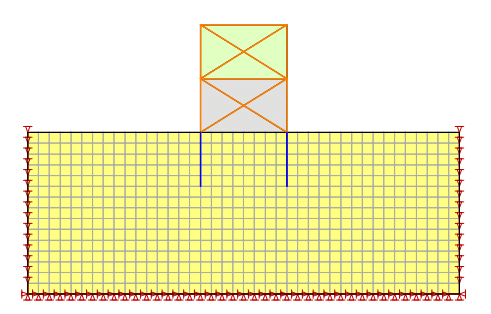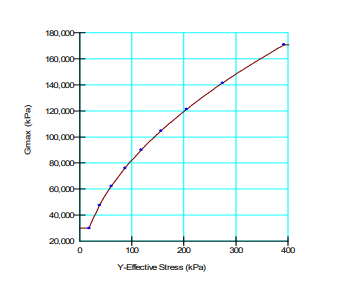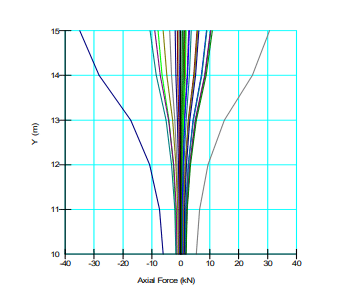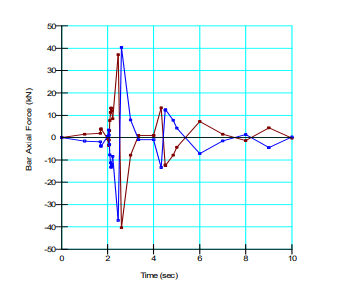## Introduction

The response of the ground can be influenced by the rigidity of a structure. Stated conversely, the presence of a stiff structure could possibly affect the response of the ground to earthquake shaking.

QUAKE/W has not been formulated with the intent of analyzing the dynamic response of structures to earthquake shaking, but QUAKE/W does have beam and bar elements, which can be used to consider the structural stiffness in a dynamic analysis.

The primary purpose of this example is to illustrate some modeling techniques and to check the behavior of beam and bar elements in a dynamic analysis.

No attempt is made here to simulate a real case. The soil and structural properties are completely arbitrary and were selected purely for illustrative purposes.

## Numerical Simulation

Figure 1 shows the problem configuration for this illustrative example. The foundation is first modeled with the Initial static analysis to determine the initial stress conditions of the foundation. The building pile foundation is then included as beams in the two shaking analyses. The building frame is modeled with structural bars.##### Figure 1. Example of including a structure in a dynamic analysis.

Structural beams and bars in QUAKE/W have no mass. Generally, the mass of the structural elements is small relative to the soil mass and, consequently, their mass is of little importance. The stiffness is extremely important, but not the mass.

In the case of a building like in this example, the mass of the building may, however, have some influence on the ground response. If this is deemed important, the mass can be included in regular elements within the frame. In the above diagram, the top floor of the building has one element within the frame. The element has been assigned a small unit weight and a small stiffness. The cross-sectional area of the element times the unit weight might be made equal to the weight of the frame. The G modulus could be set to a small number, so that the shear in the elements does not absorb a significant amount of the energy.

Analyses are included for a case without any mass in the frame, and a case with some mass in the frame. All analyses include a Linear Elastic material for the foundation with a total unit weight of 20kN/m3 and a Damping Ratio of 0.1. The Gmax function for the foundation soil is shown in Figure 2.##### Figure 2. Foundation Gmax function.

When mass is included in the frame, the total unit weight is 1 kN/m3 with a constant Gmax of 1,000 kPa. The earthquake record for both shaking analyses is shown in Figure 3.## Results and Discussion

Figure 4 illustrates the axial force variations in the piles during the earthquake with the building mass included. These forces do not include the static forces – they are only the dynamic forces.##### Figure 4. Axial force in the piles during the shaking.

Figure 5 shows a plot of the axial forces in the two lower-floor cross members. Note how they change from tension (negative) to compression and how they have opposing tension and compression, as they properly should.##### Figure 5. Axial dynamic forces in the cross members in the lower floor.

Remember that structural elements in QUAKE/W do not include the mass of the beams and bars – only the stiffness and rigidity.

## Summary and Conclusions

QUAKE/W is not intended for analyzing the dynamic behavior of structures for the purpose of designing the structure itself. Estimates of the building rigidity can, however, be included in an earthquake ground response analysis.

This example illustrates some modeling techniques used to include the effects of a structure, including the mass of the structure, in a QUAKE/W analysis.
This example also demonstrates that the structural elements in QUAKE/W function in accordance with expectations.